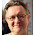Saturday, January 18, 2014

The Power of Zero

----------------------------------------- I recently received a few links (, , , ) which show some 'brain-melting maths' to prove that the infinite series represented by S = 1 + 2 + 3 + 4 + 5 + ... is equal to a small negative fraction (- 1⁄12 to be specific). I am sure that the brain melting preceded the maths rather than the other way around, else this would not have seen the light of day, let alone so many posts. On the face of it, the proof looks to be quite smart, which explains why it is showing up in so many places. Plus, the honorific attached to the authors was that of Professors, so very few people actually challenged it. The rebuttal  missed the point as well. First a summary: The 'proof' uses the Cesaro summation of the Grandi's series as the foundation (*NOTHING* wrong with this, in the context). Reusing the text from the 'Bad Rebuttal' which actually provides a good summary: ============= Begin Ctrl-c-Ctrl-v ==================== We'll consider three infinite series: S1 = 1 - 1 + 1 - 1 + 1 - 1 + ... S2 = 1 - 2 + 3 - 4 + 5 - 6 + ... S3 = 1 + 2 + 3 + 4 + 5 + 6 + ... S1 is something called Grandi's series. According to the video, taken to infinity, Grandi's series alternates between 0 and 1. So to get a value for the full series, you can just take the average - so we'll say that S1 =  1⁄2. <snip> Now, consider S2. We're going to add S2 to itself. When we write it, we'll do a bit of offset: S2          : 1 - 2 + 3 - 4 + 5 - 6 + ... + S2      :      1 - 2 + 3 - 4 + 5 + ... ====   : =============================== 2 * S2     : 1 - 1 + 1 - 1 + 1 - 1 + ... So 2 * S2 = S1; therefore S2 = S1⁄2= 1⁄4. Now, let's look at what happens if we take the S3, and subtract S2 from it: S3           :    1 + 2 + 3 + 4 + 5 + 6 + ... - S2         : - [1 - 2  + 3 - 4  + 5  - 6 + ...] ===== : =============================== S3 - S2    :    0 + 4 + 0 + 8 + 0 + 12 + ... or S3 - S2     : = 4(1 + 2 + 3 + ...) = 4 * S3 and therefore -S2 = 3 * S3 and S3 = -1⁄12. ======== End Ctrl-c-Ctrl-v and Bad-Maths ========== Good Maths - The actual rebuttal, etc: First, there is nothing wrong with assigning the Cesaro summation of 1⁄2 to Grandi's series, as long as you know 'where you came from'. Understand it this way, if a car starts at rest, and then accelerates at a constant rate to hit 1 mile per hour (a rather safe driver) in exactly one hour, and then decelerates at the same constant rate in the next hour, his speed at the hour mark would be 1, then 0, then 1 again and so on. This is what you expect at the end of each term in the Grandi series (n1:1, n2:1-1=0, n3:1-1+1=1, and so on). By following the above approach, the car would have covered 1⁄2 mile in the first hour, and another 1⁄2 mile in the second. If the driver continues in the same fastidious vein till gas stocks last, he would have done an 'average' of 1⁄2 mile per hour over the course of the adventure. You can also think of this as the area under the line graph that keeps going from 0 to 1 and then back to 0 after each unit on the x-axis. Phil Plait uses a similar Stairways to Heaven analogy in link  - look at that graph for reference or the first graph on the Google Spreadsheet  [http://goo.gl/jtbZi2].S2 derivation is also correct as the average converges to 14:

Now that we have the Cesaro sums out of the way, we are ready to identify the fallacy - remember the power of the zero!
I think Ramanujan was pranking Dr Hardy in 1913 with this. Cheers, Rahul Raj Not-a-mathematician-in-spite-of-my-teachers'-best-efforts Links: Bad Maths:  Authors' site - 'Original' source : http://www.numberphile.com/videos/analytical_continuation1.html  On YouTube - Same source: http://www.youtube.com/watch?v=w-I6XTVZXww#t=22  Authors' blog where they defend the same : http://periodicvideos.blogspot.co.uk/2014/01/thanks.html  Bad Astronomer - Phil Plait not understanding the less celestial numbers/ the FB link I received through multiple sources: http://www.slate.com/blogs/bad_astronomy/2014/01/17/infinite_series_when_the_sum_of_all_positive_integers_is_a_small_negative.html Bad Rebuttal:  Someone who 'misses' the point : http://scientopia.org/blogs/goodmath/2014/01/17/bad-math-from-the-bad-astronomer/ Almost Good Maths:  Calculations for the Cesaro sum - Google Docs link: http://goo.gl/jtbZi2 Wiki:  http://en.wikipedia.org/wiki/1_%2B_2_%2B_3_%2B_4_%2B_⋯

1.Update : [Jan 19,2014 19:30 IST - GMT+05:30]
Phil Plait (who had one of the most popular versions of this story) has retracted his endorsement, but IMHO, he misses the point as well at http://www.slate.com/blogs/bad_astronomy/2014/01/18/follow_up_the_infinite_series_and_the_mind_blowing_result.html

He has also questioned the Cesaro sum for S2 whereas if you follow the spreadsheet http://goo.gl/jtbZi2 , you will appreciate that 1/4 captures the *impact* of S2 quite well.

Could someone tell Phil (@BadAstronomer), that he needs to retract parts of the retraction now :) ?

Thanks.

PS: I understand Cesaro sum as the *impact* of a series, and not the value/ sum of it

2.I completely disagree with this stupid post

1.Umm.. why? Unless it is on account of the Calvin-Answer-Phenomenon (http://www.gocomics.com/calvinandhobbes/1986/01/07 - 2+7=___?)

2.The comment contains nothing but emotion, unless it is selfreferential, in which case it is redundant. Thanks Rajul Raj for clear thinking!

3.Ashutosh (Chaos), I think this is for you.

Unless it was for me :duh:

3.Neat logical illusion, no?

1.Exactly! Some people took it as a mathematical proof, instead of the parlour trick it actually is!